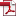• KSII Transactions on Internet and Information Systems
Monthly Online Journal (eISSN: 1976-7277)

# Speed-up of the Matrix Computation on the Ridge Regression

Vol. 15, No. 10, October 31, 202110.3837/tiis.2021.10.001, Download Paper (Free):#### Abstract

Artificial intelligence has emerged as the core of the 4th industrial revolution, and large amounts of data processing, such as big data technology and rapid data analysis, are inevitable. The most fundamental and universal data interpretation technique is an analysis of information through regression, which is also the basis of machine learning. Ridge regression is a technique of regression that decreases sensitivity to unique or outlier information. The time-consuming calculation portion of the matrix computation, however, basically includes the introduction of an inverse matrix. As the size of the matrix expands, the matrix solution method becomes a major challenge. In this paper, a new algorithm is introduced to enhance the speed of ridge regression estimator calculation through series expansion and computation recycle without adopting an inverse matrix in the calculation process or other factorization methods. In addition, the performances of the proposed algorithm and the existing algorithm were compared according to the matrix size. Overall, excellent speed-up of the proposed algorithm with good accuracy was demonstrated.